Acceleration Plot PropertyManager

The Define Acceleration Plot PropertyManager allows you to plot acceleration results for linear and nonlinear dynamic studies.

To display this PropertyManager, run a dynamic study. Right-click Results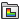and select Define Acceleration Plot.

Display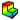Component Select an acceleration componentAX: X Acceleration AY: Y Acceleration AZ: Z Acceleration ARES: Resultant Acceleration BX: X Angular Acceleration (shell studies only) BY: Y Angular Acceleration (shell studies only) BZ: Z Angular Acceleration (shell studies only) Directions are based on the global coordinate system.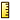Units Select a unit for the acceleration plot.

 Show as vector plot Generates a vector plot where a vector is plotted at each node to show the magnitude and direction of the selected component. You can control the size and density of the vectors in a vector plot using the Vector Plot Options PropertyManager. Show PSD value When checked, the PSD values are shown for a particular solution step. Otherwise, the RMS values across the whole frequency range are shown.

Plot Step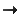Plot for Single Step Select this option to plot the results at the selected solution step.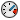Displays the time corresponding to the selected solution step.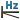Displays the frequency corresponding to the selected plot step. Available for harmonic and random vibration studies.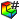Plot Step Sets the solution step number for the plot.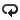Plot Bounds across All Steps Select this option to plot extreme values regardless of the solution step they occur at. Select one of the following:
 Maximum Displays the maximum algebraic values (envelope plot) across all solution steps. Minimum Displays the minimum algebraic values (envelope plot) across all solution steps. Absolute maximum Displays the absolute maximum values (envelope plot ) across all solution steps.
Acceleration results are available for nodes only.

Deformed Shape

If checked, the selected acceleration component is plotted on the deformed shape of the model.

 Automatic Displays the default Scale Factor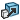that the program uses to scale the largest deformation to 10% of the largest dimension of the smallest box that surrounds the model. True scale Displays the actual deformed shape of the model (Scale Factor is 1.0). User defined Allows you to enter your own Scale Factor. In most cases, a larger scale factor helps to appreciate the deflection.

Property

 Include title text Type a custom title for the plot. Associate plot with name view orientation Associate a predefined view orientation with the active plot.